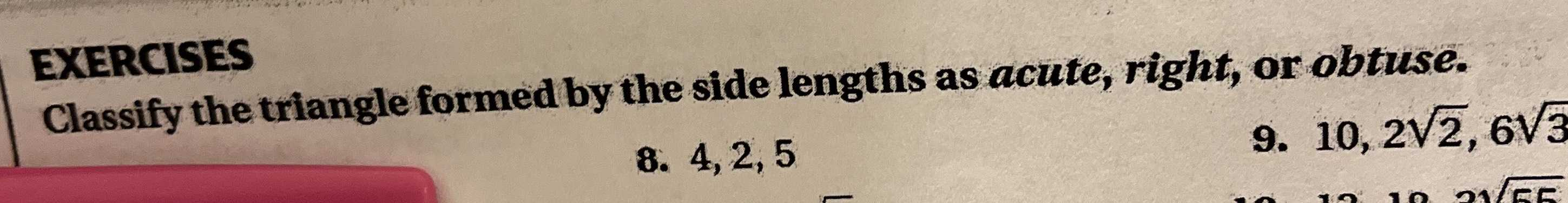### Still have math questions?

Trigonometry
QuestionEXERCISES Classify the triangle formed by the side lengths as acute, right, or obtuse.

Obtuse Scalene Triangle Side $$a = 2$$ Side $$b = 4$$ Side $$c = 5$$ Angle $$\angle A = 22.332 ^ { \circ } = 22 ^ { \circ } 19 ^ { \prime } 54 " = 0.38976 rad$$

Angle $$\angle B = 49.458 ^ { \circ } = 49 ^ { \circ } 27 ^ { \prime } 30 ^ { \prime \prime } = 0.86321 rad$$

Angle $$\angle C = 108.21 ^ { \circ } = 108 ^ { \circ } 12 ^ { \prime } 36 ^ { \prime \prime } = 1.88862 rad$$

Area $$= 3.79967$$

Perimeter $$p = 11$$

Semiperimeter $$s = 5.5$$

Solution
View full explanation on CameraMath App.